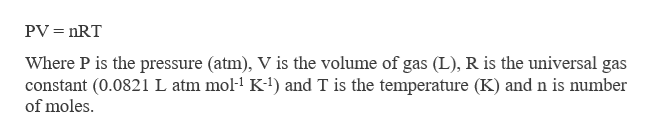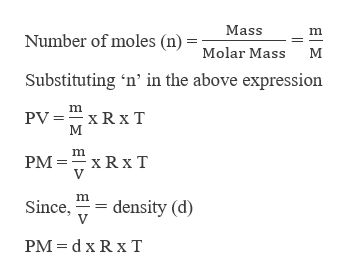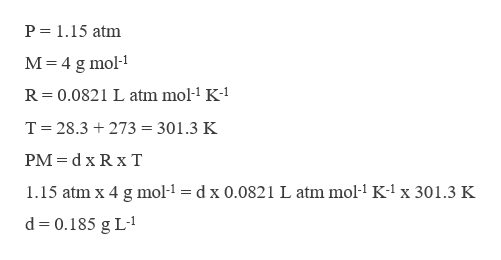# Calculate the density of helium in a helium balloon at 28.3°C. (Assume that the pressure inside the balloon is 1.15 atm.)

Question
14 views

Calculate the density of helium in a helium balloon at 28.3°C. (Assume that the pressure inside the balloon is 1.15 atm.)

check_circle

Step 1

The pressure inside the balloon is given as 1.15 atm and the temperature of Helium balloon is 28.3 oC. The molar mass of He is 4 g. The density can be calculated as,help_outlineImage TranscriptionclosePV nRT Where P is the pressure (atm), V is the volume of gas (L), R is the universal gas constant (0.0821 L atm mol-1 K-1) and T is the temperature (K) andn is number of moles. fullscreen
Step 2

Using the above expression, the density can be derived as,help_outlineImage TranscriptioncloseMass Number of moles (n) Molar Mass М Substituting 'n' in the above expression m PV =xR x T М m PM xRx T V m. Since, density (d) PM d x Rx T fullscreen
Step 3

Substituting the values in the a...help_outlineImage TranscriptioncloseP 1.15 atm M 4 g mol1 R 0.0821 L atm mol-1 K-1 T 28.3 273 = 301.3 K PM d x Rx T 1.15 atm x 4 g mol1 = d x 0.0821 L atm mol-1 K-lx 301.3 K d 0.185 g L-1 fullscreen

### Want to see the full answer?

See Solution

#### Want to see this answer and more?

Solutions are written by subject experts who are available 24/7. Questions are typically answered within 1 hour.*

See Solution
*Response times may vary by subject and question.
Tagged in

### Gas laws